## Chapter 5. Data Representation and Storage

This chapter shows how different data types are represented in storage and describes how the CF90 and MIPSpro 7 Fortran 90 compilers use storage.

Numbers shown on the formats are bit positions, which represent powers of 2 in binary notation. Code that depends on internal representation is not portable and might not conform with the Fortran standard.Note:: Storage words are represented here with bits counted from the right, making bit 0 the low-order bit and bit 31 or 63 the high-order bit. This agrees with the convention used in the integer-type bit functions as well as the convention used in Cray hardware documentation. It does not agree with some conventions used in some other UNICOS and UNICOS/mk software documentation.

This chapter describes the machine representation of data. The last sections in this chapter describe storage issues, including overindexing.

## Data Representation for UNICOS Systems

The following sections describe the representation of data on UNICOS systems, including CRAY T90 systems that support Cray floating-point arithmetic. These subsections do not describe data representation on CRAY T90 systems that support IEEE floating-point arithmetic. For information pertaining to CRAY T90 systems that support IEEE floating-point arithmetic, see “Data Representation for CRAY T90 Systems That Support IEEE Floating-point Arithmetic”.

### Integer Type

All integer data is 64 bits (KIND=8), 2's complement.

When slower integer operations (f90 -O nofastint) are in effect, the range for INTEGER(KIND=8) operations is -263 < I < 263 or approximately -1018 < I < 1018.

When fast integer operations (f90 -O fastint) are in effect, which is the default, the range for INTEGER(KIND=8) operations is -246 < I < 246 or approximately -1013 < I < 1013.

Figure 5-1. Default 64-bit integers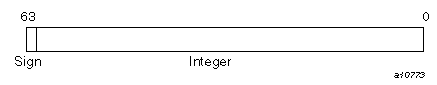Figure 5-2. Fast integer operations with INTEGER(KIND=8), UNICOS systems (except CRAY T90 systems that support IEEE floating-point arithmetic)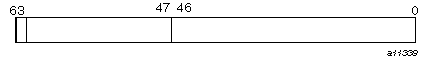To declare an entity to be of type integer, specify one of the following:

• KIND=1, KIND=2, KIND=4, or KIND=8.

• KIND=KIND(kind_expr), where kind_expr is a scalar initialization expression with a kind type parameter that evaluates to 1, 2, 4, or 8.

### Real Type

Real (floating-point) numbers are represented in a packed representation of a binary mantissa and an exponent (power of 2). The bits in a Cray word are used to represent a real number as follows:

Figure 5-3. Real type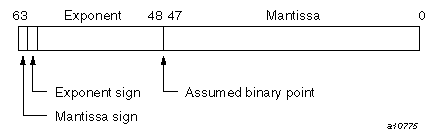Notes on real data type representation:

The exponent is a power of 2, represented by a number that is 400008 higher than the actual value; this is called a bias. The effect of the bias is that the second bit in the word serves as the exponent's sign bit. This bit's usage is the inverse of the mantissa's sign bit, as follows:

Bit

Applies to

1 value indicates

63

Mantissa

Negative

62

Exponent

Positive

The exponent is represented by the second through sixth digits in an octal printout; these digits have the range 40000 through 577768 for a positive exponent, and 37777 through 200038 for a negative exponent.

When the bias is accounted for, the range of all exponents is as follows (notice the negative range is one smaller):

• 2-17775 to 217776 (octal)

or

• 2-8189 to 28190 (decimal)

The mantissa is a 48-bit signed fraction. The sign of the mantissa is separated from the rest of the mantissa as shown in the preceding diagram. The mantissa is not complemented for negative values. That is, the mantissa for -10.0 is the same as for +10.0.

In terms of decimal values, the floating-point format of the CPU allows representation of numbers to about 15 significant decimal digits in the following approximate decimal range:

.367 × 10-2465 < R < .273 × 10 2466

A zero value is not biased and is represented as a word of all zeros.

Following are some sample numbers as represented within memory:

 Decimal Octal Hexadecimal 10.0 040004500000000000000 4004A00000000000 -10.0 140004500000000000000 C004A00000000000 0.1 0377756314631463146315 3FFDCCCCCCCCCCCD -0.1 1377756314631463146315 BFFDCCCCCCCCCCCD

Figure 5-4. Binary version of 10.0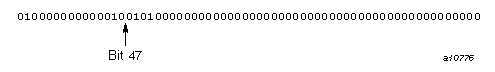The leftmost bit, with a 0 value, indicates a positive mantissa; that is, the real value is positive. The next bit, set to 1, is the sign bit of the exponent, indicating a positive exponent value; that is, the absolute value of the number is 1.0 or greater. The value 4 in the exponent (100 appearing to the left of bit 47) means that the binary fraction in the mantissa is multiplied by 24 (or, to express it another way, the binary point is moved 4 bits to the right from the highest bit of the mantissa.) Interpreted in this way, the first 4 digits of the mantissa, 1010, indicate the real decimal value 10.0. You can display other values by printing them with formats O22, Z16, or B64.

To declare an entity to be of type real, specify one of the following:

• KIND=4, KIND=8

• KIND=KIND(kind_expr), where kind_expr is a scalar initialization expression with a kind type parameter that evaluates to 4 or 8

Note that a real data object with KIND=4 has the same internal representation as a real data object with KIND=8. Numeric inquiry functions on a real data object with KIND=4 return different values than on a real data object with KIND=8. A numeric operation on a real data object with KIND=4 returns the same result as the same numeric operation on a real data object with KIND=8.

#### Normalized Floating-point Numbers

A nonzero, floating-point number is normalized if the most significant bit of the mantissa is nonzero. This condition implies that the mantissa has been shifted as far left as possible and the exponent adjusted accordingly. Therefore, the floating-point number has no leading zeros in the mantissa. The exception is that a normalized floating-point zero is all zeros.

When your program creates a floating-point number by inserting an exponent of 400608 into a KIND=8 integer word, you should normalize the result before using it in a floating-point operation. To do this, add the unnormalized floating-point operand to 0. Compiler optimization suppresses an operation such as X=X+0. You can perform it with code such as the following:

 ```DATA REALZERO /0./ X = X + REALZERO```

### Double-precision Type

A double-precision value is represented by 2 words. The first has the same format as the real type. The second word uses bits 0 through 47 as 48 additional bits of the mantissa. The other 16 bits of the second word must be zeros. Double-precision numbers can be in the following range:

• 2-8188 .LE. R < 28189

or approximately

• .367 × 10-2465 < R < .273 × 102466

Figure 5-5. Double-precision type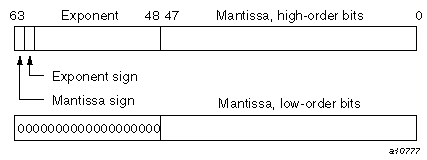To declare an entity to be of type double precision, specify one of the following:

• REAL(KIND=16).

• REAL(KIND=KIND(kind_expr)), where kind_expr is a scalar initialization expression with a kind type parameter that evaluates to 16.

### Single-precision Complex Type

A single-precision complex value is represented by 2 words, each of which has the same format as the real type. The first word represents the real part, and the second represents the imaginary part. Each word has the same range as a real value.

Figure 5-6. Single-precision complex type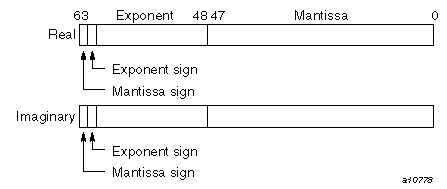To declare an entity to be of single-precision complex type, specify one of the following:

• KIND=4 or KIND=8.

• KIND=KIND(kind_expr), where kind_expr is a scalar initialization expression that evaluates to 4 or 8.

Note that a complex data object with KIND=4 has the same internal representation as a complex data object with KIND=8. Numeric inquiry functions on a complex data object with KIND=4 return different values than on a complex data object with KIND=8. A numeric operation on a complex data object with KIND=4 returns the same result as the same numeric operation on a complex data object with KIND=8.

### Double-precision Complex Type

Values of double precision complex type are represented by 4 words. The first 2 words are the real part, and the last 2 words are the imaginary part. The real part and the imaginary part each have the same range as a double precision value.

Figure 5-7. Double-precision complex type (real portion)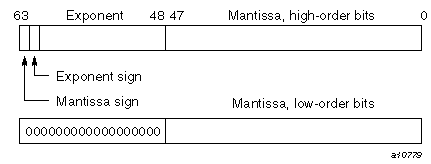Figure 5-8. Double-precision complex type (imaginary portion)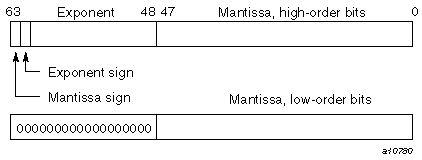To declare an entity to be of double-precision complex type, specify one of the following:

• KIND=16.

• KIND=KIND(kind_expr), where kind_expr is a scalar initialization expression with a kind type parameter that evaluates to 16.

### Character Type

Characters are represented by 8-bit ASCII codes packed eight per word.

Figure 5-9. Character type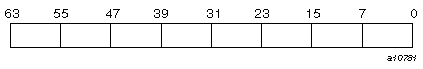The CF90 compiler does not support a nondefault character type. The only kind value supported is 1.

### Logical Type

A logical variable uses one 64-bit word. Its value is true if the numeric value in the word is negative (typically, -1), and it is false if the numeric value in the word is nonnegative (typically, 0).Note:: Silicon Graphics does not guarantee a particular internal representation of logical values on any machine or system; the CF90 compiler is designed on the assumption that logical values will be used only as described in the Fortran standard. Therefore, it is not good programming practice to exploit gaps in type checking, such as between a function reference and its function value, to use logical values as numbers or vice versa.

To declare an entity to be of logical type, you can specify one of the following:

• KIND=1, KIND=2, KIND=4, or KIND=8.

• KIND=KIND(kind_expr), where kind_expr is a scalar initialization expression with a kind type parameter that evaluates to 1, 2, 4, or 8.

Note that logical entities with KIND=1, KIND=2, KIND=4, and KIND=8 all occupy 64 bits.

### Cray Character Pointers

Cray character pointers include a word address, bit offset, and bit length field.

Figure 5-10. 64-bit addressing for UNICOS systems (except CRAY T90 systems)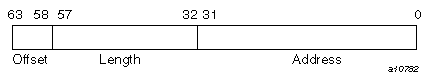Figure 5-11. 64-bit addressing for CRAY T90 systems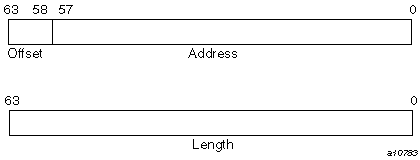## Data Representation for IRIX systems

The following sections describe the representation of data on IRIX systems.Note:: On IRIX systems, KIND=4 values are stored in 32 bits and can be packed two per word.

### Integer Type

The following sections describe integer data representation of KIND=1, 2, 4, and 8 on IRIX systems.

#### KIND=1

Range: -27 < I < 27 or approximately -102 < I < 102

Figure 5-12. INTEGER(KIND=1) on IRIX systems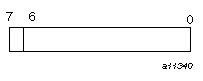To declare 8-bit integers, specify one of the following:

• KIND=1.

• KIND=KIND(kind_expr), where kind_expr is a scalar initialization expression with a kind type parameter that evaluates to 1.

#### KIND=2

Range: -215 < I < 215 or approximately -104 < I < 104

Figure 5-13. INTEGER(KIND=2) on IRIX systems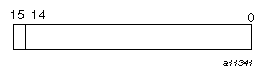To declare 16-bit integers, specify one of the following:

• KIND=2.

• KIND=KIND(kind_expr), where kind_expr is a scalar initialization expression with a kind type parameter that evaluates to 2.

#### KIND=4

Range: -231 < I < 231 or approximately -109 < I < 109

Figure 5-14. INTEGER(KIND=4) on IRIX systems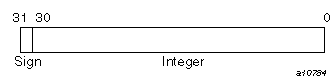To declare 32-bit integers, specify one of the following:

• KIND=4.

• KIND=KIND(kind_expr), where kind_expr is a scalar initialization expression with a kind type parameter that evaluates to 4.

#### KIND=8

Range: -263 < I < 263 or approximately -1018 < I < 1018

Figure 5-15. INTEGER(KIND=8) on IRIX systems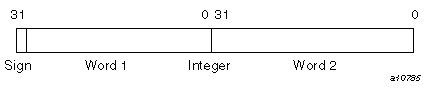To declare 64-bit integers, specify one of the following:

• KIND=8.

• KIND=KIND(kind_expr), where kind_expr is a scalar initialization expression with a kind type parameter that evaluates to 8.

### Real Type

The following sections describe real data representation of KIND= 4, 8, and 16 on IRIX systems. Real (floating-point) numbers are represented in a packed representation of a sign, an exponent (power of 2), and a binary mantissa.

#### KIND=4

Range: -2-125 .LE. I < 2128 or approximately -10-38 .LE. I < 1038

Figure 5-16. REAL(KIND=4) on IRIX systems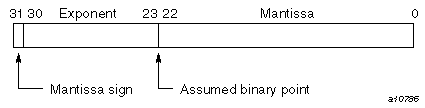To declare 32-bit reals, specify one of the following:

• KIND=4.

• KIND=KIND(kind_expr), where kind_expr is a scalar initialization expression with a kind type parameter that evaluates to 4.

Notes on real data type representation:

The exponent is a power of 2, represented by a number that is 1778 higher than the actual value; this is called a bias. The effect of the bias is that the second bit in the word serves as the exponent's sign bit. This bit's usage is the inverse of the mantissa's sign bit, as follows:

 Bit Applies to 1 value indicates 31 Mantissa Negative 30 Exponent Positive ( > 0 )

The exponent is represented by the second through ninth digits in a binary printout; these digits have the range 011111112 through 111111102 for a positive exponent, and 000000002 through 011111102 for a negative exponent.

When the bias is accounted for, the range of all exponents is as follows:

• 2-177 to 2177 (octal)

or

• 2-127 to 2127 (decimal)

The mantissa is a 24-bit fraction with an assumed leading 1; that is, the leading 1 is not stored. The only exception is for the value 0, which has an assumed leading 0. The sign of the mantissa is separated from the rest of the mantissa as shown in the preceding diagram. The mantissa is not complemented for negative values. That is, the mantissa for -10.0 is the same as for +10.0.

In terms of decimal values, the 32-bit floating-point format allows representation of numbers to about 7 significant decimal digits in the following approximate decimal range:

1.18 × 10-38 < R < 3.4 × 1038

A zero value is not biased and is represented as a word of all zeros.

The following are some sample numbers as represented within memory:

Decimal

Octal

10.0

010110000000

41200000

-10.0

030110000000

C1200000

0.1

007563146315

3DCCCCCD

-0.1

027563146315

BDCCCCCD

Figure 5-17. Binary version of 10.0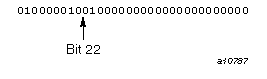The leftmost bit, with a 0 value, indicates a positive mantissa; that is, the real value is positive. The next 8 bits (10000010, or decimal 130) are the exponent. Subtracting the bias of 127 yields an exponent of 3, meaning that the binary fraction in the mantissa is multiplied by 23. To express it another way, the binary point is moved 3 bits to the right from the mantissa's highest bit. Interpreted this way, the first 4 bits of the mantissa, 010, indicate the real decimal value 10.0 (remember that there is an assumed 1 to the left of the mantissa in the IEEE floating-point format with a binary point to its immediate right). You can display other values by printing them with formats O11, Z8, or B32.

#### KIND=8

Double precision, REAL(KIND=8), values are represented in 2 words on IRIX systems.

Range: -2-1021 .LE. I < 21024 or approximately -10-308 .LE. I < 10308

Figure 5-18. REAL(KIND=8) on IRIX systems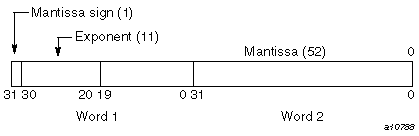To declare 64-bit reals, specify one of the following:

• KIND=8.

• KIND=KIND(kind_expr), where kind_expr is a scalar initialization expression with a kind type parameter that evaluates to 8.

#### KIND=16

Quad precision, REAL(KIND=16), values are represented in 4 words on IRIX systems. For more information on quad precision representation IRIX systems, see math(3m).

Range: -2-967 .LE. I < 21023 or approximately -10-292 .LE. I < 10308

Figure 5-19. REAL(KIND=16) on IRIX systems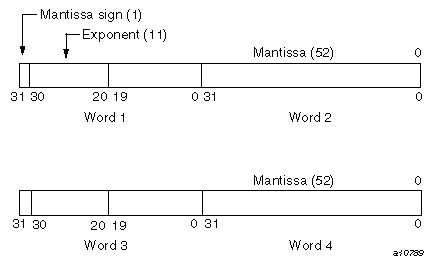To declare 128-bit reals, specify one of the following:

• KIND=16.

• KIND=KIND(kind_expr), where kind_expr is a scalar initialization expression with a kind type parameter that evaluates to 16.

### Complex Type

The following sections describe complex data representation of KIND=4, 8, and 16 on IRIX systems. A complex value has two parts. The first part represents the real part, and the second represents the imaginary part. Each word has the same range as a real value.

#### KIND=4

A single-precision, KIND=4, complex value is represented by 2 words. The first word represents the real part, and the second represents the imaginary part. Each word has the same range as a real value.

Range: -2-125 .LE. I < 2128 or approximately -1038 .LE. I < 1038

Figure 5-20. COMPLEX(KIND=4) on IRIX systems (real portion)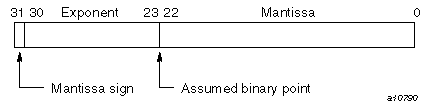Figure 5-21. COMPLEX(KIND=4) on IRIX systems (imaginary portion)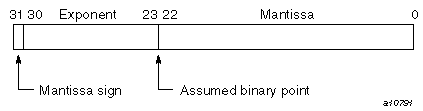To declare an entity to be of single-precision, complex type, specify one of the following:

• KIND=4.

• KIND=KIND(kind_expr), where kind_expr is a scalar initialization expression with a kind type parameter that evaluates to 4.

#### KIND=8

A double-precision, KIND=8, complex value is represented by 4 words. The first 2 words represent the real part, and the second 2 words represent the imaginary part. Each word has the same range as a real value.

Range: -2-1021 .LE. I < 21024 or approximately -10308 .LE. I < 10308

Figure 5-22. COMPLEX(KIND=8) on IRIX systems (real portion)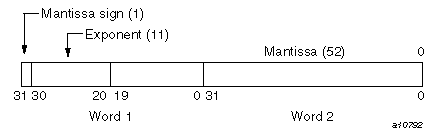Figure 5-23. COMPLEX(KIND=8) on IRIX systems (imaginary portion)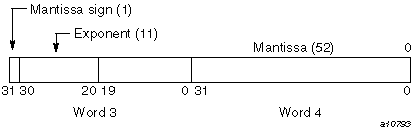To declare an entity to be of double-precision, complex type, specify one of the following:

• KIND=8.

• KIND=KIND(kind_expr), where kind_expr is a scalar initialization expression with a kind type parameter that evaluates to 8.

#### KIND=16

A quad precision, KIND=16, complex value is represented by 8 words. The first 4 words represent the real part, and the second 4 words represent the imaginary part. Each word has the same range as a real value.

Range: -2-967 .LE. I < 21023 or approximately -10-292 .LE. I < 10308

Figure 5-24. COMPLEX(KIND=16) on IRIX systems (real portion)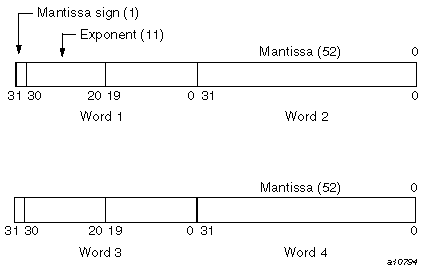Figure 5-25. COMPLEX(KIND=16) on IRIX systems (imaginary portion)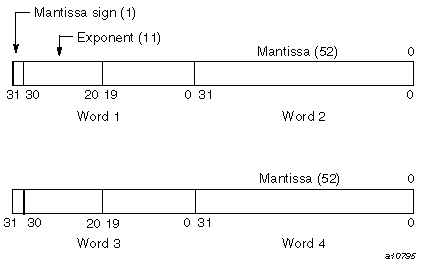To declare an entity to be of quad precision, complex type, specify one of the following:

• KIND=16.

• KIND=KIND(kind_expr), where kind_expr is a scalar initialization expression with a kind type parameter that evaluates to 16.

### Character Type

Characters are represented by 8-bit ASCII codes. On IRIX systems, the codes are stored in 1 byte.

Figure 5-26. Character type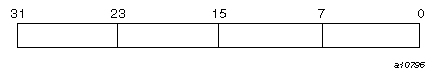The MIPSpro 7 Fortran 90 compiler does not support a nondefault character type. The only kind value supported is 1.

### Logical Type

Logical entities specified as KIND=1, KIND=2, and KIND=4 occupy 32 bits on IRIX systems. Logical entities specified as KIND=8 occupy 64 bits on IRIX systems. Its value is true if the numeric value in the word is one (1). Its value is false if the numeric value in the word is zero (0).Note:: Silicon Graphics does not guarantee a particular internal representation of logical values on any machine or system; the MIPSpro 7 Fortran 90 compiler is designed on the assumption that logical values will be used only as described in the Fortran standard. Therefore, it is not good programming practice to use logical values as numbers or vice versa.

To declare an entity to be of logical type, you can specify one of the following:

• KIND=1, KIND=2, KIND=4, or KIND=8.

• KIND=KIND(kind_expr), where kind_expr is a scalar initialization expression with a kind type parameter that evaluates to 1, 2, 4, or 8.

### Cray Character Pointers (Deferred Implementation)

Cray character pointers include a byte address and a byte length field.

Figure 5-27. 32-bit addressing on IRIX systems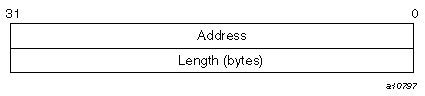## Data Representation for UNICOS/mk Systems

The following sections describe the representation of data on UNICOS/mk systems.Note:: On UNICOS/mk systems, KIND=4 values are stored in 32 bits and can be packed two per word.

### Integer Type

The following subsections describe integer data representation of KIND=1, 2, 4, and 8 on UNICOS/mk systems.

#### KIND=1, KIND=2, or KIND=4

Range: -231 < I < 231 or approximately -109 < I < 109

Figure 5-28. Integer KIND=1, 2, or 4 on UNICOS/mk systems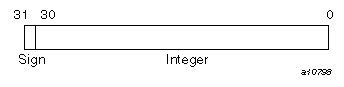To declare 32-bit integers, specify one of the following:

• KIND=1, KIND=2, or KIND=4.

• KIND=KIND(kind_expr), where kind_expr is a scalar initialization expression with a kind type parameter that evaluates to 1, 2, or 4.

#### KIND=8

Range: -263 < I < 263 or approximately -1018 < I < 1018

Figure 5-29. INTEGER(KIND=8) on UNICOS/mk systems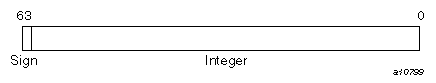To declare 64-bit integers, specify one of the following:

• KIND=8.

• KIND=KIND(kind_expr), where kind_expr is a scalar initialization expression with a kind type parameter that evaluates to 8.

### Real Type

The following sections describe real data representation of KIND=4 and 8. Real (floating-point) numbers are represented in a packed representation of a sign, an exponent (power of 2), and a binary mantissa.

#### KIND=4

Range: -2-125 .LE. I < 2128 or approximately -10-38 .LE. I < 1038

Figure 5-30. REAL(KIND=4) on UNICOS/mk systems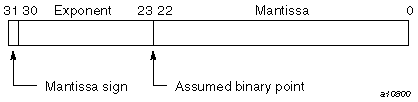To declare 32-bit reals, specify one of the following:

• KIND=4.

• KIND=KIND(kind_expr), where kind_expr is a scalar initialization expression with a kind type parameter that evaluates to 4.

Notes on real data type representation:

The exponent is a power of 2, represented by a number that is 1778 higher than the actual value; this is called a bias. The effect of the bias is that the second bit in the word serves as the exponent's sign bit. This bit's usage is the inverse of the mantissa's sign bit, as follows:

 Bit Applies to 1 value indicates 31 Mantissa Negative 30 Exponent Positive ( > 0 )

The exponent is represented by the second through ninth digits in a binary printout; these digits have the range 011111112 through 111111102 for a positive exponent, and 000000002 through 011111102 for a negative exponent.

When the bias is accounted for, the range of all exponents is as follows:

• 2-177 to 2177 (octal)

or

• 2-127 to 2127 (decimal)

The mantissa is a 24-bit fraction with an assumed leading 1; that is, the leading 1 is not stored. The only exception is for the value 0, which has an assumed leading 0. The sign of the mantissa is separated from the rest of the mantissa as shown in the preceding diagram. The mantissa is not complemented for negative values. That is, the mantissa for -10.0 is the same as for +10.0.

In terms of decimal values, the 32-bit floating-point format allows representation of numbers to about 7 significant decimal digits in the following approximate decimal range:

1.18 × 10-38 < R < 3.4 × 1038

A zero value is not biased and is represented as a word of all zeros.

The following are some sample numbers as represented within memory:

Decimal

Octal

10.0

010110000000

41200000

-10.0

030110000000

C1200000

0.1

007563146315

3DCCCCCD

-0.1

027563146315

BDCCCCCD

Figure 5-31. Binary version of 10.0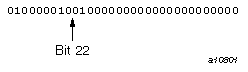The leftmost bit, with a 0 value, indicates a positive mantissa; that is, the real value is positive. The next 8 bits (10000010, or decimal 130) are the exponent. Subtracting the bias of 127 yields an exponent of 3, meaning that the binary fraction in the mantissa is multiplied by 23; to express it another way, the binary point is moved 3 bits to the right from the mantissa's highest bit. Interpreted this way, the first 4 bits of the mantissa, 010, indicate the real decimal value 10.0; remember that there is an assumed 1 to the left of the mantissa in the IEEE floating-point format with a binary point to its immediate right. You can display other values by printing them with formats O11, Z8, or B32.

#### KIND=8

Range: -2-1021 .LE. I < 21024 or approximately -10-308 .LE. I < 10308

Figure 5-32. REAL(KIND=8) on UNICOS/mk systems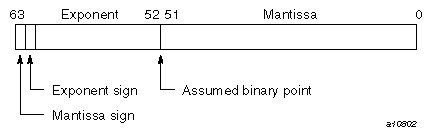To declare 64-bit reals, specify one of the following:

• KIND=8.

• KIND=KIND(kind_expr), where kind_expr is a scalar initialization expression with a kind type parameter that evaluates to 8.

### Complex Type

The following sections describe complex data representation of KIND=4 and KIND=8 on UNICOS/mk systems. A complex value has two parts. The first part represents the real part, and the second represents the imaginary part. Each word has the same range as a real value.

#### KIND=4

A KIND=4 complex value consists of 2 parts. The first part represents the real portion, and the second represents the imaginary portion. Each part has the same range as a 32-bit (or KIND=4) real value.

Range: -2-125 .LE. I < 2128 or approximately -10-38 .LE. I <1038

Figure 5-33. COMPLEX(KIND=4) on UNICOS/mk systems (real portion)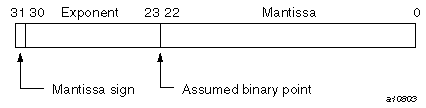Figure 5-34. COMPLEX(KIND=4) on UNICOS/mk systems (imaginary portion)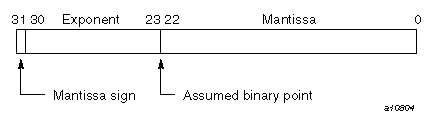To declare an entity to be of complex type with a total length of 64 bits, specify one of the following:

• KIND=4.

• KIND=KIND(kind_expr), where kind_expr is a scalar initialization expression with a kind type parameter that evaluates to 4.

#### KIND=8

A single-precision, KIND=8, complex value is represented by 2 words. The first word represents the real part, and the second represents the imaginary part. Each word has the same range as a 64-bit (or KIND=8) real value.

Range: -2-1021 .LE. I < 21024 or approximately -10-308 .LE. I < 10308

Figure 5-35. COMPLEX(KIND=8) on UNICOS/mk systems (real portion)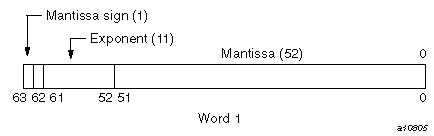Figure 5-36. COMPLEX(KIND=8) on UNICOS/mk systems (imaginary portion)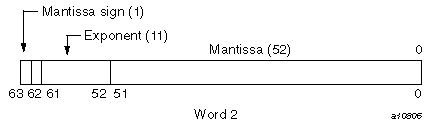To declare an entity to be of single-precision, complex type, specify one of the following:

• KIND=8.

• KIND=KIND(kind_expr), where kind_expr is a scalar initialization expression with a kind type parameter that evaluates to 8.

### Character Type

Characters are represented by 8-bit ASCII codes. On UNICOS/mk systems, the codes are packed 8 per word.

Figure 5-37. Character type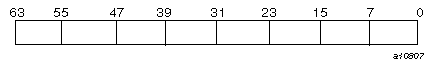The CF90 compiler does not support a nondefault character type. The only kind value supported is 1.

### Logical Type

A logical variable uses one word. Its value is true if the numeric value in the word is nonzero, and it is false if the numeric value in the word is zero.Note:: Silicon Graphics does not guarantee a particular internal representation of logical values on any machine or system; the CF90 compiler is designed on the assumption that logical values will be used only as described in the Fortran standard. Therefore, it is not good programming practice to use logical values as numbers or vice versa.

To declare an entity to be of logical type, you can specify one of the following:

• KIND=1, KIND=2, KIND=4, or KIND=8.

• KIND=KIND(kind_expr), where kind_expr is a scalar initialization expression with a kind type parameter that evaluates to 1, 2, 4, or 8.

On UNICOS/mk systems, all KIND=1, 2, and 4 occupy 32 bits. The KIND=8 specification occupies 64 bits.

### Cray Character Pointers

Cray character pointers include a byte address and a byte length field. On UNICOS/mk systems, character pointers are 128-bit objects, as follows:

Figure 5-38. Cray character pointers on UNICOS/mk systems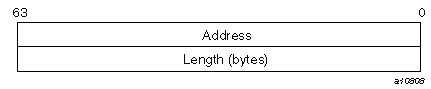## Data Representation for CRAY T90 Systems That Support IEEE Floating-point Arithmetic

The following sections describe the representation of data on CRAY T90 systems that support IEEE floating-point arithmetic.

### Integer Type

The following sections describe integer data representation of KIND=1, 2, 4, and 8 on CRAY T90 systems that support IEEE floating-point arithmetic.

#### KIND=1, KIND=2, or KIND=4

Range: -231 < I < 231 or approximately -109 < I < 109

Figure 5-39. Integer KIND=1, 2, or 4 on CRAY T90 systems that support IEEE floating-point arithmetic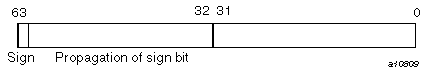To declare 32-bit integers, specify one of the following:

• KIND=1, KIND=2, or KIND=4.

• KIND=KIND(kind_expr), where kind_expr is a scalar initialization expression with a kind type parameter that evaluates to 1, 2, or 4.

#### KIND=8

By default, the range for INTEGER(KIND=8) operations is -263 < I < 263 or approximately -1018 < I < 1018. When fast integer operations are specified on the f90(1) command line, the range for INTEGER(KIND=8) operations is -250 < I < 250 or approximately -1015 < I < 1015.

Figure 5-40. Default INTEGER(KIND=8) on CRAY T90 systems that support IEEE floating-point arithmetic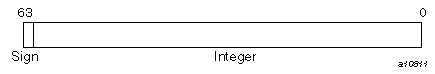Figure 5-41. Fast operations with INTEGER(KIND=8) on CRAY T90 systems that support IEEE floating-point arithmetic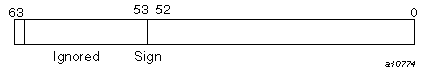To declare 64-bit integers, specify one of the following:

• KIND=8.

• KIND=KIND(kind_expr), where kind_expr is a scalar initialization expression with a kind type parameter that evaluates to 8.

### Real Type

The following sections describe real data representation of KIND=4, 8, and 16 on CRAY T90 systems that support IEEE floating-point arithmetic. Real (floating-point) numbers are represented in a packed representation of a sign, an exponent (power of 2), and a binary mantissa.

#### KIND=4 and KIND=8

Range: -2-1021 .LE. I < 21024 or approximately -10-308 .LE. I < 10308

Figure 5-42. Real KIND=4 or 8 on CRAY T90 systems that support IEEE floating-point arithmetic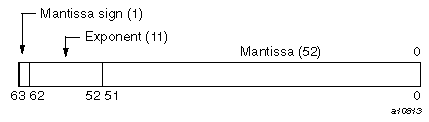To declare 64-bit reals, specify one of the following:

• KIND=4 or KIND=8.

• KIND=KIND(kind_expr), where kind_expr is a scalar initialization expression with a kind type parameter that evaluates to 4 or 8.

For additional information on how real data is represented on CRAY T90 systems that support IEEE floating-point arithmetic, see "Notes on real data type representation" in “KIND=4”. The information presented there for UNICOS/mk systems applies to CRAY T90 systems that support IEEE floating-point arithmetic.

Note that a real data object with KIND=4 has the same internal representation as a real data object with KIND=8. Numeric inquiry functions on a real data object with KIND=4 return different values than on a real data object with KIND=8. A numeric operation on a real data object with KIND=4 returns the same result as the same numeric operation on a real data object with KIND=8.

#### KIND=16

Double precision, REAL(KIND=16), values are represented in 2 words on CRAY T90 systems that support IEEE floating-point arithmetic.

Range: -2-16381 .LE. I < 216384 or approximately -10-4932 .LE. I < 104932

Figure 5-43. REAL(KIND=16) on CRAY T90 systems that support IEEE floating-point arithmetic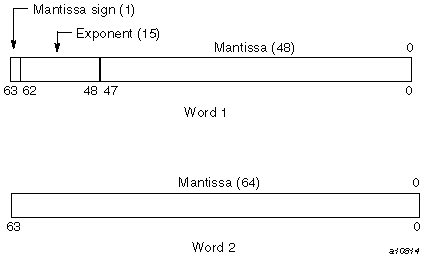To declare 64-bit reals, specify one of the following:

• KIND=16.

• KIND=KIND(kind_expr), where kind_expr is a scalar initialization expression with a kind type parameter that evaluates to 16.

### Complex Type

The following sections describe complex data representation of KIND=4, 8, and 16 on CRAY T90 systems that support IEEE floating-point arithmetic. A complex value has two parts. The first part represents the real part, and the second represents the imaginary part. Each word has the same range as a real value.

#### KIND=4 and KIND=8

A single-precision, KIND=4 or KIND=8, complex value is represented by 2 words. The first word represents the real part, and the second represents the imaginary part. Each word has the same range as a real value.

Range: -2-1021 .LE. I < 21024 or approximately -10-308 .LE. I < 10308

Figure 5-44. Complex KIND=8 or 4 on CRAY T90 systems that support IEEE floating-point arithmetic (real portion)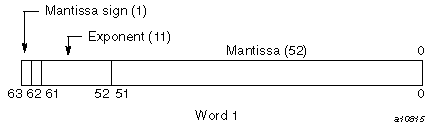Figure 5-45. Complex KIND=8 or 4 on CRAY T90 systems that support IEEE floating-point arithmetic (imaginary portion)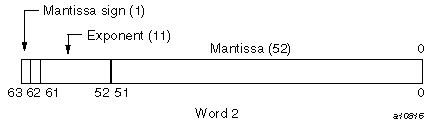To declare an entity to be of single-precision, complex type, specify one of the following:

• KIND=4 or KIND=8.

• KIND=KIND(kind_expr), where kind_expr is a scalar initialization expression with a kind type parameter that evaluates to 4 or 8.

Note that a complex data object with KIND=4 has the same internal representation as a complex data object with KIND=8. Numeric inquiry functions on a complex data object with KIND=4 return different values than on a complex data object with KIND=8. A numeric operation on a complex data object with KIND=4 returns the same result as the same numeric operation on a complex data object with KIND=8.

#### KIND=16

A double-precision, KIND=16, complex value is represented by 4 words. The first two words represent the real part, and the second two words represent the imaginary part. Each word has the same range as a real value.

Range: -2-16381 .LE. I < 216384 or approximately -10-4932 .LE. I < 104932

Figure 5-46. COMPLEX(KIND=16) on CRAY T90 systems that support IEEE floating-point arithmetic (real portion)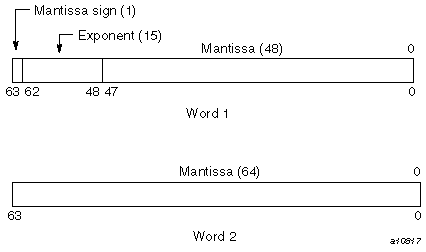Figure 5-47. COMPLEX(KIND=16) on CRAY T90 systems that support IEEE floating-point arithmetic (imaginary portion)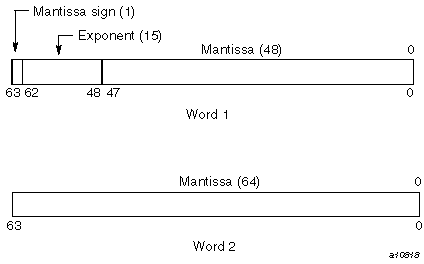To declare an entity to be of double-precision, complex type, specify one of the following:

• KIND=16.

• KIND=KIND(kind_expr), where kind_expr is a scalar initialization expression with a kind type parameter that evaluates to 16.

### Character Type

Characters are represented by 8-bit ASCII codes. The codes are packed 8 per word.

Figure 5-48. Character type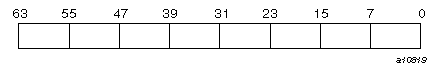The CF90 compiler does not support a nondefault character type. The only kind value supported is 1.

### Logical Type

A logical variable uses one word. Its value is true if the numeric value in the word is nonzero, and it is false if the numeric value in the word is zero.Note:: Silicon Graphics does not guarantee a particular internal representation of logical values on any machine or system; the CF90 compiler is designed on the assumption that logical values will be used only as described in the Fortran standard. Therefore, it is not good programming practice to use logical values as numbers or vice versa.

To declare an entity to be of logical type, you can specify one of the following:

• KIND=1, KIND=2, KIND=4, or KIND=8.

• KIND=KIND(kind_expr), where kind_expr is a scalar initialization expression with a kind type parameter that evaluates to 1, 2, 4, or 8.

On CRAY T90 systems that support IEEE floating-point arithmetic, all KIND=1, KIND=2, and KIND=4 specifications occupy 32 bits. The KIND=8 specification occupies 64 bits.

### Cray Character Pointers

Cray character pointers are two words in length. The first word includes an offset and an address. The second word includes the byte length field.

Figure 5-49. Cray character pointer for CRAY T90 systems that support IEEE floating-point arithmetic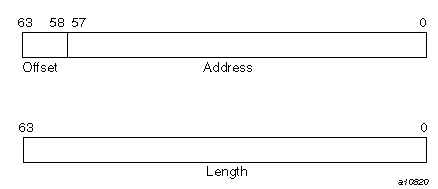## Storage Issues

This section describes how the CF90 and MIPSpro 7 Fortran 90 compilers use storage, including how these compilers accommodate programs that use overindexing.Note:: The information in this section assumes that you are using the default data representations. On UNICOS/mk systems, specifying -i 32 or -s default32 on the f90(1) command line changes the storage and data representation of all noncharacter data types. This affects data that is storage sequence-associated. Mixing data types is not recommended when these command line options are used. On IRIX systems, the following options to the f90(1) command affect storage and data representation:-d16 changes default double precision and double complex to 128 bits -i4 changes default integer and logical to 32 bits -i8 changes default integer and logical to 64 bits -n32 and -64 change pointer sizes and the maximum amount of addressable memory -r4 changes default real and complex to 32 bits/64 bits -r8 changes default real and complex to 64 bits/128 bits

### Storage Units and Sequences

A numeric storage unit can be one of the following:

• A word on UNICOS and UNICOS/mk systems of 64 bits.

• A word on IRIX systems of 32 bits.

A character storage unit is an 8-bit byte.

A storage sequence is a contiguous group of storage units with a consecutive series of addresses. Each array and each common block is stored in a storage sequence. The size of a storage sequence is the number of storage units it contains. Two storage sequences are associated if they share at least one storage unit.

All nondefault types have an unspecified storage unit. The -s default32 option on the f90(1) command line changes the number of bits in a numeric storage unit for UNICOS/mk systems. There is no longer a relationship between storage units after this command line option is used.

The following list shows the storage units for the default types on UNICOS systems:

 Type Storage units Integer 1 Real (single precision) 1 Real (double precision) 2 Complex 2 Logical 1

Complex values occupy twice the storage of real values. The real portion of the complex value occupies the first half of the total storage; the imaginary portion of the complex value occupies the second half of the total storage, as follows:

• On UNICOS and UNICOS/mk systems, a double precision or complex value (KIND=4 or KIND=8) uses a storage sequence of two numeric storage units. The first storage unit contains the most significant bits of a double-precision value or the real part of a complex value. The second storage unit contains the least significant bits of a double-precision value or the imaginary part of a complex value. Double precision and double complex data types are not supported on UNICOS/mk systems.

On IRIX systems, a double-precision value uses a storage sequence of 8 or 16 bytes. Depending on the KIND= specification, a complex value uses 8, 16, or 32 bytes. The first half of the bytes used contains the most significant bits of a double-precision value or the real part of a complex value. The last half of the bytes used contains the least significant bits of a double-precision value or the imaginary part of a complex value.

• On UNICOS and UNICOS/mk systems, a double-complex value occupies 4 words of storage; the first 2 words contain the real part of the complex value, and the second 2 words contain the imaginary part.

On IRIX systems, a double-complex value occupies 16 bytes of storage; the first 8 bytes contain the real part of the complex value, and the second 8 bytes contain the imaginary part.

On IRIX systems, a quad precision complex value occupies 32 bytes of storage; the first 16 bytes contain the real part of the complex value, and the second 16 bytes contain the imaginary part.

A character value is represented as an 8-bit ASCII code, packed 8 characters per word on UNICOS and UNICOS/mk systems; this value is packed 4 characters per byte on IRIX systems. The storage size depends on the length specification of the value.Note: The Fortran standard does not specify the relationship between storage units and computer words, and it does not specify any relation between default numeric and character storage units.

### Static and Stack Storage

With static storage, any variable that is allocated memory occupies the same address throughout program execution. Allocation is determined before program execution.

Code using static storage can be used with Autotasking, multitasking, and macrotasking if variables in static storage conform to the following guidelines:

• Loops are Autotasked regardless of the presence of variables in static or stack storage. Scoping is controlled by the presence of PRIVATE or SHARED parameters on the DOALL Autotasking directive. If a subroutine that contains static data is called from within an autotasked loop, static data is treated as shared data, which means that the static data must be protected by GUARD and ENDGUARD Autotasking directives.

• Variables in static storage can be read when loops are multitasked and macrotasked. If a loop modifies variables in static storage, you must use guards (GUARD and ENDGUARD Autotasking directives) or locks (LOCKON() and LOCKOFF() calls) to protect the variables.

For more information on Autotasking directives, see the CF90 Commands and Directives Reference Manual, or the MIPSpro 7 Fortran 90 Commands and Directives Reference Manual. For more information on locks, see the LOCKON(3f) or LOCKOFF(3f) man pages.

Stack storage is the default storage allocation for the CF90 compiler on UNICOS and UNICOS/mk systems. On IRIX systems, stack storage is the MIPSpro 7 Fortran 90 default for all subprograms, but static storage is the default for items that require 256 bits of storage in a main program. The stack is an area of memory where storage for variables is allocated when a subprogram or procedure begins execution. These variables are released when execution completes. The stack expands and contracts as procedures are entered and exited. Autotasking and recursion require a stack.

When stack storage is used, the value of a variable is not saved between invocations of a subprogram unless it is specified in a SAVE or DATA statement. When f90 -e v (UNICOS and UNICOS/mk systems) or f90 -static (IRIX systems) is specified, all user variables are treated as if they appeared in a SAVE statement. When -e v or -static is in effect, compiler-generated temporary variables and the calling sequence are still allocated to the stack.Note:: If f90 -e i is specified, variables are reset for each invocation of a subprogram, even in static storage. Therefore, the SAVE or DATA statement is necessary to preserve the value of a variable between invocations. This information applies only to UNICOS and UNICOS/mk systems.

The way in which the amount of memory available for the stack is determined depends on your platform. On UNICOS and UNICOS/mk systems, it is determined by the STACK directive, available with the segldr(1) or cld(1) loaders; see the segldr(1) or cld(1) man pages for more information. On IRIX systems, you can use the limit(1) command to change the amount of stack space that a program is allowed; see the limit(1) man page for more information.

A heap is memory that, like a stack, is dynamically allocated; it is used internally.

The CF90 and MIPSpro 7 Fortran 90 compilers allocate variables to storage according to the following criteria:

• Variables in common blocks are always allocated in the order in which they appear in COMMON statements.

• Data in modules are statically allocated.

• User variables that are defined or referenced in a program unit, and that also appear in SAVE or DATA statements, are allocated to static storage, but not necessarily in the order shown in your source program.

• Other referenced user variables are assigned to the stack. If -e v (UNICOS and UNICOS/mk systems) or -static (IRIX systems) is specified on the f90(1) command line, referenced variables are allocated to static storage. This allocation does not necessarily depend on the order in which the variables appear in your source program.

• Compiler-generated variables are assigned to a register or to memory (to the stack or heap), depending on how the variable is used. Compiler-generated variables include DO-loop trip counts, dummy argument addresses, temporaries used in expression evaluation, argument lists, and variables storing adjustable dimension bounds at entries.

• Automatic objects may be allocated to either the stack or to the heap, depending on how much stack space is available when the objects are allocated.

• Heap or stack allocation can be used for TASK COMMON variables and some compiler-generated temporary data such as automatic arrays and array temporaries.Note:: Unreferenced user variables not appearing in COMMON statements are not allocated.

### Dynamic Memory Allocation (UNICOS Systems Only)

Many FORTRAN 77 programs contain a memory allocation scheme that expands an array in a common block located in central memory at the end of the program. This practice of expanding a blank common block or expanding a dynamic common block (sometimes referred to as overindexing) causes conflicts between user management of memory and the dynamic memory requirements of UNICOS libraries. It is recommended that you modify programs rather than expand blank common blocks, particularly when migrating from other environments.

Figure 5-50, shows the structure of a program under the UNICOS operating system in relation to expanding a blank common block. In both figures, the user area includes code, data, and common blocks.

Figure 5-50. Memory use under UNICOS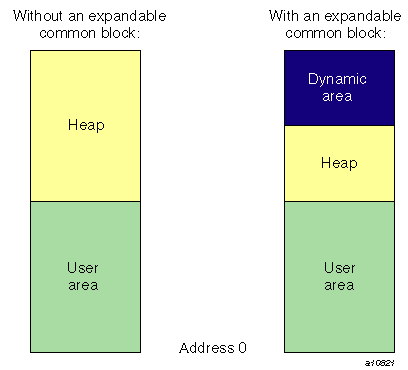There are two ways to change your code. The standard method, shown in “Changing Your Code: Standard Method” is preferred.

#### Changing Your Code: Standard Method

You can use the ALLOCATE statement to dynamically allocate an array. Use the following three-step process:

1. For arrays that expand in a common block, define Fortran allocatable arrays in a Fortran module.

2. Replace the common block definition in all source files that use the global array with a USE statement.

3. Use the ALLOCATE statement in place of any calls to the MEMORY routine.

Original code:

 ``` PROGRAM TEST C Puts array X in blank common: COMMON X(1) ... C Adds 100000 words to blank common: CALL MEMORY ('UC',100000) ... DO 10, I=1,100000 X(I) = RANF() 10 CONTINUE ...```

Converted code (after steps 1 and 2):

 ``` MODULE GLOBAL_DATA ! STEP 1 REAL, SAVE, ALLOCATABLE :: X(:) END MODULE ... PROGRAM TEST USE GLOBAL_DATA ! STEP 2 LIMIT = 100000 ALLOCATE (X(LIMIT)) ! STEP 3 ... DO 10 I = 1,LIMIT X(I) = RANF() 10 CONTINUE ... END```

#### Changing Your Code: Nonstandard Method

The nonstandard way to change your program is by using the following two-step process:

1. For arrays that expand in a common block, define Cray pointers that point to the first address in each array.

2. Change any calls to memory to calls to library routine HPALLOC(3).

Original code:

 ``` PROGRAM TEST C Puts array X in blank common: COMMON X(1) ... C Adds 100000 words to blank common: CALL MEMORY ('UC',100000) ... DO 10, I=1,100000 X(I) = RANF() 10 CONTINUE ...```

Converted code (after steps 1 and 2):

 ``` PROGRAM TEST COMMON /WORK/ IPTR ... C Establish array location at runtime: POINTER (IPTR,X(1)) ... C Effective common block size: CALL HPALLOC (IPTR,100000,ERRCODE,0) ... DO 10 I=1,100000 X(I) = RANF() 10 CONTINUE ...```